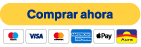top of page###### Logistic regressions

This bootcamp is an invitation to use logistic regressions and statistical tests for binary classification problems

1. What is machine learning and logistic regressions?

2. A look at R.

3. Formal description of the logistic regressions.

4. Implementation of logistic regressions to detect plausibility of reviews.

5. Advanced aspects of logistic regressions.

Syllabus

1. Introduction

• What is machine learning and logistic regressions

• Mathematical notions and probability elements

• Probability elements

2. Installation of R, R Studio and Packages required for Ridge Regression

• Installation of R and Rstudio

• For Windows

• For Mac

• For Ubuntu

• For other versions of Linux

• Installing R Packages

3. Logistic regression

• Maximum likelihood

• Binary classification and logistic regression

• Geometric and probabilistic interpretation

4. Favorable review classifier

5. Selected Topics in Linear Regressions